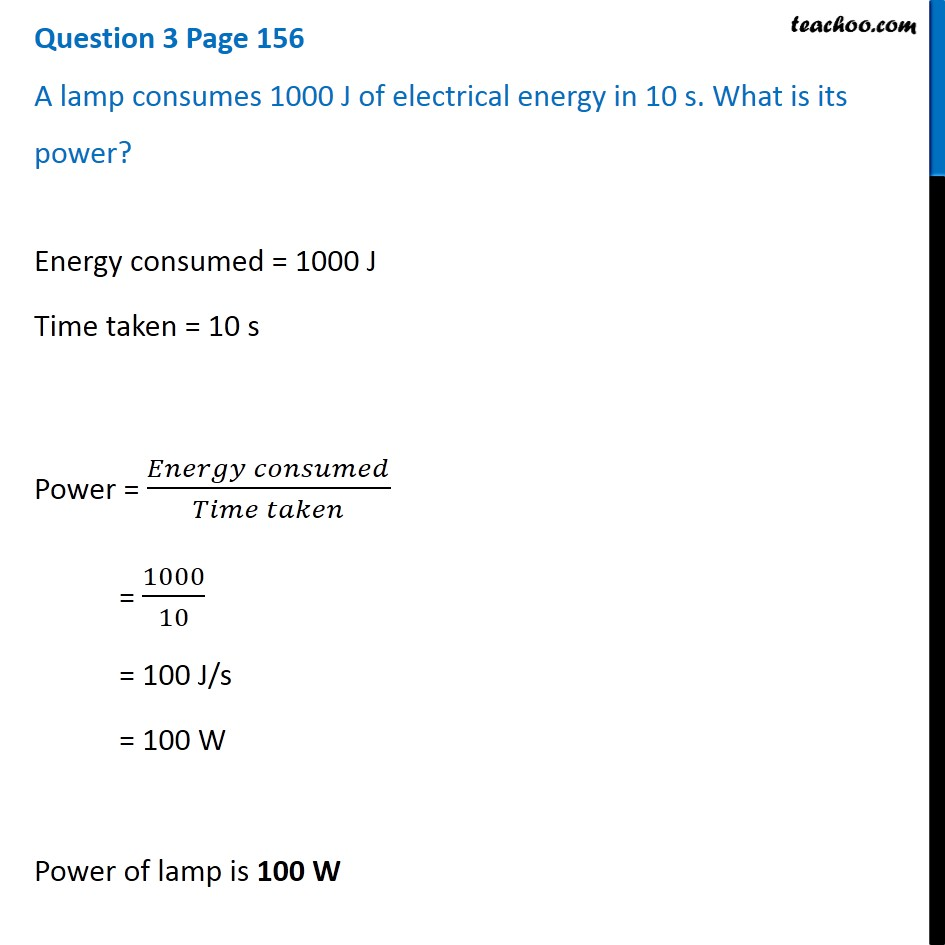Questions from Inside the chapter

Class 9
Chapter 11 Class 9 - Work and EnergyGet live Maths 1-on-1 Classs - Class 6 to 12

### Transcript

Question 3 Page 156 A lamp consumes 1000 J of electrical energy in 10 s. What is its power? Energy consumed = 1000 J Time taken = 10 s Power = (𝐸𝑛𝑒𝑟𝑔𝑦 𝑐𝑜𝑛𝑠𝑢𝑚𝑒𝑑)/(𝑇𝑖𝑚𝑒 𝑡𝑎𝑘𝑒𝑛) = 1000/10 = 100 J/s = 100 W Power of lamp is 100 W